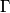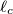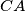IMO Shortlist 2011 problem G8

Kvaliteta:
Avg: 0,0
Težina:
Avg: 9,5
Let$ABC$ be an acute triangle with circumcircle$\Gamma$. Let$\ell$ be a tangent line to$\Gamma$, and let$\ell_a, \ell_b$ and$\ell_c$ be the lines obtained by reflecting$\ell$ in the lines$BC$,$CA$ and$AB$, respectively. Show that the circumcircle of the triangle determined by the lines$\ell_a, \ell_b$ and$\ell_c$ is tangent to the circle$\Gamma$.

Proposed by Japan
Izvor: Međunarodna matematička olimpijada, shortlist 2011